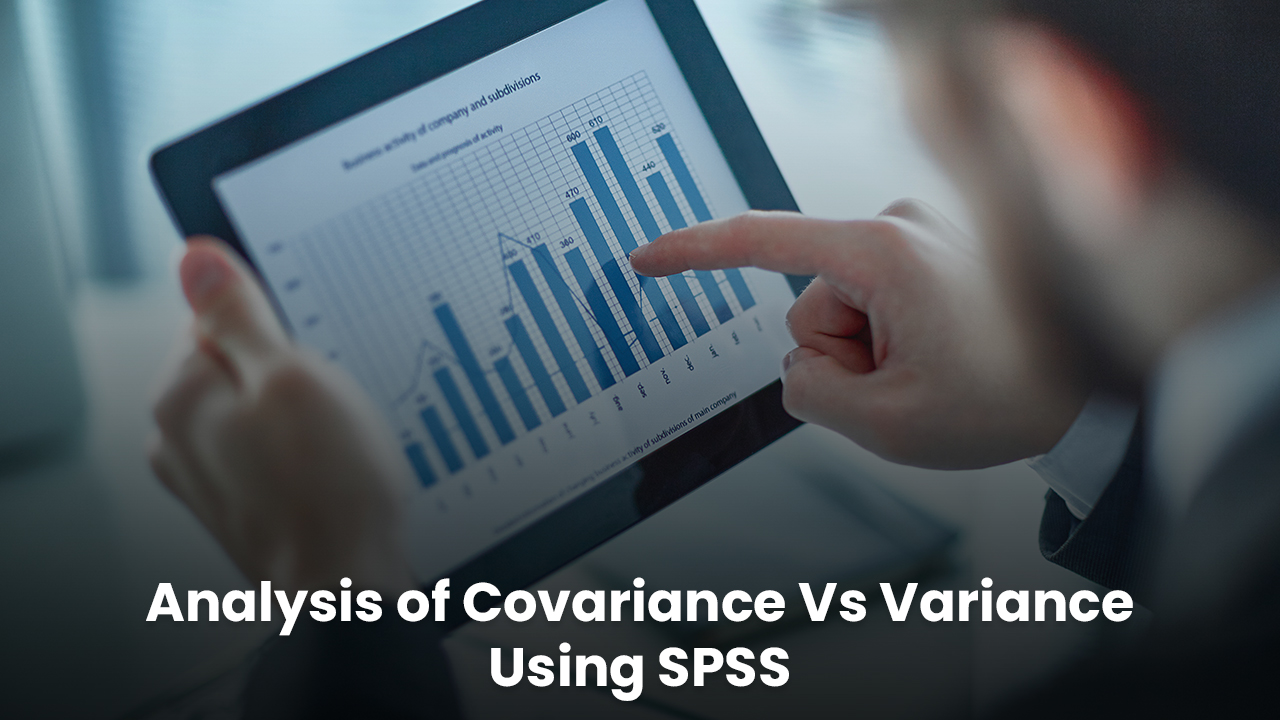# Difference between Analysis of Covariance (ANCOVA) & (ANOVA)## Definition of ANOVA

ANOVA is extended as an analysis of variance that is described as a statistical technique to determine the means of two populations. The amount of variation between the samples is bifurcated while some specific causes of dependency are found out.

## Classification of ANOVA

1. One-way ANOVA: One factor investigates the difference factor among the available categories with a possibility of having n number of values.
2. Two-way ANOVA: Two factors are included in the investigation process simultaneously to measure analysis of the influential variable

## Definition of ANCOVA

It stands for analysis of covariance, which is an extended form of ANOVA. The elimination of effects is done before research can be conducted. ANOVA and regression analysis is an important working point considering the variability of all other variables. Key differences between ANOVA and ANCOVA work into the statistical components that are taken into consideration.

## Differences between the chosen parameters

Analysis of covariance is applicable to a comparison of one variable with other variables present in the population while considering other variables. ANOVA is on the other hand used for comparing and contrasting more than two populations. ANCOVA is used in research, where the effects of some antecedent variables are removed. As an example pre-test scores can be defined which are used as covariates in pre-test and post-test experimental designs. In the case of ANOVA, the process helps in finding out the difference between groups of data that are statistically significant. Levels of variance include group samples taken from each of the components.

One-way ANOVA is used for the determination of statistically significant differences that is applicable for more than three independent groups. One-way ANOVA is the independent samples of t-tests that compare the means for two groups. Two-way ANOVA is used only when one measurement variable is working on two of the nominal variables. In simple words, while an experiment gets quantitative outcomes, there are two categorical explanatory variables presented that work on the appropriateness of ANOVA. For example, social media can be taken as an independent variable, and groups are assigned to low, medium, and high levels of social media users to find out if there exists any difference in sleeping hours per night.

Different types of ANOVA include the use of Multivariate of analysis (MANOVA) which differs from ANOVA as the multiple dependent variables simultaneously assess the only dependent variable at a time. The p-value is the area that associates with a probability of observing a result as big as the one that is obtained from experiment F0. The null hypothesis seems to be true since the inferential statistic method concludes a population of a given sample set. The value which is calculated from the data given to a researcher is called the statistics or f-value. Now, in case a calculated f-value is found to be larger than the F critical value then the null hypothesis can be rejected easily. Three-way ANOVA has also been identified as effective in finance and social sciences. Comparison can be conducted based on the ANOVA uses, inclusion, and covariate functionalities.

Comparison factors ANOVA ANCOVA
Definition The examination is conducted to reach an outcome for multiple groups of data. ANCOVA is identified as a technique that is applicable for the removal of metric-scale dependent variables before undertaking research.
Covariate It is ignored in this case The consideration is gained
WG variation Attributes are associated with WG variation looking after individual differences Group variation is divided into individual differences.
Application Used in both linear and non-linear models Used only in the linear model
BG variation Attributes between BG variations exist that work as a barrier to the treatment of within-group (WG) variation Individual differences and covariates worked within a group of WG variations.The technique of identifying variance among the means of multiple groups works on homogeneity. It is a part of variance that is used for taking off the impact within more than one metric-scaled undesirable variable. ANOVA can be used for calculating the linear and nonlinear model components. On the other hand, Analysis of covariance (ANCOVA) uses a single linear model. ANOVA characterizes group variations that are exclusive to treatment. ANCOVA divides between the group variation in treatment and covariates. ANOVA exhibits individual differences while the group variance individual differences are assigned to the components within regression analysis.

## Conclusion

ANOVA SPSS is associated with large amounts of data processing in statistical analysis. Like the t-tests, are conducted for finding the differences between groups of data whichever is statistically significant. A covariate is identified as an independent variable that is looked upon to manage the "effects of the categorical independent on an interval dependent variable", which thereafter works on the covariate control. T-tests are an identified method that determines whether different populations are statistically different from each other or not. In this entire process, ANOVA and ANCOVA come handy within the SPSS data analysis software.